# Chapter 8. Priority queues

The Priority Queue ADT is designed for systems that maintaining a collection of prioritized elements, where elements are removed from the collection in order of their priority. Traditionally, we think of priorities as being numbers, with lower numbers representing higher priorities; priority-one elements, for example, are removed before priority-two elements. The Priority Queue ADT includes four operations:

• `add(x)` to add `x` into the priority queue.

• `isEmpty()` to query whether the priority queue is empty.

• `remove()` to remove the highest-priority element from the priority queue, returning it.

• `peek()` to query what the highest-priority element of the priority queue is.

Priority queues turn up in several applications. A simple application comes from processing jobs, where we process each job based on how urgent it is. For example, busy dry cleaners may ask each customer when the job must be done, and then they may choose to clean articles of clothing in the order of how little time is left for each article. For that matter, some college students complete assignments based on which is due first. In a similar way, operating systems often use a priority queue for the ready queue of processes to run on the CPU. (The last chapter mentioned that a queue could be used for this purpose, but in fact sophisticated operating systems place priorities on the processes.) Important programs, like those that the user is interacting with, receive a high priority; lower priority is accorded to less urgent tasks like checking periodically for new e-mail or rearranging files on disk for more efficient access. As a result, the operating system works on the background tasks only when the user is not interacting with the computer. We will see some other applications of priority queues later in this chapter.

## 8.1. Simple priority queue implementations

In using priority queues, one of the problems we confront is how a program can specify the priorities of the queue's elements. The `java.util` package does this with the `Comparable` interface: Each element has a `compareTo` method for comparing to another element. This `compareTo` method should return a negative number if the element should come before the parameter in the priority queue.

One of the simplest techniques for implementing the PriorityQueue interface is to use an unordered list, as illustrated in the ListPQueue class of Figure 8.1. The `add` method can simply add the element onto the end of the list, and the `remove` method would go through all elements to determine the minimum, removing and returning that from the list. Whether we use an ArrayList or a LinkedList, the `add` method would take O(1) time and `remove` would take O(n) time.

Figure 8.1: Implementing a priority queue using an unordered list.

```public class ListPQueue<E> {     private ArrayList<E> elts;     public ListPQueue()      { elts = new ArrayList<E>(); }     public boolean isEmpty() { return elts.size() == 0;   }     public void add(E value) { elts.add(value);           }     public E peek() {         E min = elts.get(0); // minimum seen so far         for(int i = 1; i < elts.size(); i++) {             Comparable<E> val = (Comparable<E>) elts.get(i);             if(val.compareTo(min) < 0) min = (E) val;         }         return min;     }     public E remove() {         E min = elts.get(0); // minimum seen so far         int minPos = 0;           // position of min within elts         for(int i = 1; i < elts.size(); i++) {             Comparable<E> val = (Comparable<E>) elts.get(i);             if(val.compareTo(min) < 0) { min = (E) val; minPos = i; }         }         elts.remove(minPos);         return min;     } } ```

Another technique provides the opposite tradeoff: Rather than store the list with no order, we can instead store it in descending order. Now the `add` method would be complex, involving locating the proper index for the inserted value and inserting it at that location. But the `remove` method would be quite fast: We simply remove the final element. Whether we use an ArrayList or a LinkedList, this sorted-list technique would take O(n) time for `add` and O(1) time for `remove`.

A very different technique uses the binary search tree instead. For example, we might use the TreeSet class that we saw in Chapter 5, which already stores its elements in order according to their `compareTo` method. The TreePQueue class defined in Figure 8.2 is such an example.

Figure 8.2: Implementing a priority queue using a TreeSet.

```public class TreePQueue<E> {     private TreeSet<E> elts;     public TreePQueue()      { elts = new TreeSet<E>();       }     public boolean isEmpty() { return elts.size() == 0;       }     public E peek()          { return elts.iterator().next(); }     public void add(E value) { elts.add(value);               }     public E remove() {         Iterator<E> it = elts.iterator();         E ret = it.next();         it.remove();         return ret;     } } ```

Since the Iterator returned by TreeSet's `iterator` method goes through elements in increasing order according to the elements' `compareTo` method, and the least among the elements has the highest priority, the highest-priority element is the first element returned by the iterator. Because TreeSet ensures that the tree is always roughly balanced, this technique supports the `add` and `remove` operations in O(log n) time.

(The TreePQueue implementation of Figure 8.2 contains a bug: With a priority queue, `add` should always add a new element, but TreeSet's `add` method adds a new element only if an equal element is not already present. We will not concern ourselves with this distinction, but it is an important bug.)

## 8.2. Heaps

A heap is an alternative data structure for supporting a priority queue. It still takes O(log n) time, but the idea is simpler than that lying beneath a red-black tree, and the implementation is typically more efficient, so people tend to use a heap for implementing the Priority Queue ADT. The idea is somewhat clever: Rather than store elements in a binary search tree, we'll store them in a tree having the following two properties.

• The heap must be a complete tree. The concept of a complete tree is easiest to explain by example; the following is the only possible structure of a complete tree for 10 nodes.In a complete tree, all nodes in the levels above the bottom two must have two children; and the next-to-last level must have all its non-leaves bunched to the left, all with children excepting the last non-leaf, which may be missing a right child.

Note that a complete tree has a maximum height of 1 + log2 n.

• The values of the tree must be in heap order; that is, every node of the heap must have a higher priority than either of its children. The following example illustrates this.Notice how every number is less than both of its children. As a result, the least number (i.e., the one with the highest priority) will always be at the root of the tree. This heap order is ambiguous: The following, for example, is also a legitimate heap with the same data.Note the contrast to binary search trees: Binary search trees have a flexible structure; but once the structure is chosen, there is no choice of how to place the values within that structure. In contrast, with heaps, the structure is fixed but the placement of values within the structure is flexible.

When we add a value to a heap, the heap would have to gain a node at the right side of the bottom level. So our addition will initially place the new value in that location; but it may not belong there, because the new value may have a higher priority than its parent. This is easy to address, though: We'll swap the new element and its parent. We can continue the process of swapping up the tree until the new value has a parent with a higher priority, or until the new value reaches the root.This process maintains the two heap properties, although the formal mathematical argument to this effect is somewhat difficult to make. Note that the addition algorithm takes O(log n) time, since the maximum possible number of swaps is the number of levels, 1 + log2 n.

The process for efficiently removing the highest-priority node (at the root) is not immediately obvious. You may be tempted to reverse the addition process: We shift all the values along the path from the root to the bottom right node up one level; after the above addition, for example, we would shift the nodes 3, 4, and 8 up, so that 3 would be at the root. This maintains the first heap property of completeness, but not the second. (In this case, the root's right child, 2, would have a higher priority than its parent.) To preserve the second property, you may be tempted to trickle values upward in place of the root; in the above example, we would place 2 into the root position, and then we'd place 5 into 2's former position. This approach, though, does not maintain the completeness restriction.

There is a simple O(log n) removal algorithm, though: We'll move the bottom right node to the root, and then we'll trickle it downward, swapping it each step with whichever child has a higher priority, until the higher-priority child is still of lesser priority than the trickling value, or until the value reaches a leaf.In implementing a heap, we could use the TreeNode class as we did with binary search trees, but there turns out to be a much more convenient representation: We can use an array, storing the values of the tree according to the level-order traversal. Below is a heap drawn in both its tree and its array representations.In such an array, the root value is in position 0, and a node at position i has its children at positions 2 i + 1 and 2 i + 2. Instead of thinking of a heap as a tree with the above two properties, we can instead think of the heap as an array in which the item at position i has a higher priority than those items at positions 2 i + 1 and 2 i + 2, if they exist. Using an array in this way significantly simplifies programming, and it leads to slightly improved efficiency.

The PriorityQueue class defined in Figure 8.3 uses this representation and implements the above-described addition and removal techniques.

Figure 8.3: Implementing a priority queue using a heap.

```public class PriorityQueue<E> {     private E[] data;     private int size;     public PriorityQueue(int capacity) {         data = (E[]) new Object[capacity];         size = 0;     }     public boolean isEmpty() {         return size == 0;     }     public E peek() {         return data;     }     public void add(E value) {         int pos = size;             // virtual position of value         int pPos = (pos - 1) / 2;   // position of parent         size++;         Comparable<E> compValue = (Comparable<E>) value;         while(pos > 0 && compValue.compareTo(data[pPos]) < 0) {             data[pos] = data[pPos]; // move parent into position             pos = pPos;             // and step upward             pPos = (pos - 1) / 2;         }         data[pos] = value;          // place value in final position     }     public E remove() {         E ret = data;         size--;                   // move last item to root,         Comparable<E> toTrickle = (Comparable<E>) data[size]; // trickle it down         data[size] = null;         int pos = 0;         while(true) {             int less = 2 * pos + 1; // determine lesser of children             if(less >= size) break;             Comparable<E> left = (Comparable<E>) data[less];             if(less + 1 < size && left.compareTo(data[less + 1]) > 0) {                 less++;             }             if(toTrickle.compareTo(data[less]) < 0) break;             data[pos] = data[less];             pos = less;         }         data[pos] = (E) toTrickle;         return ret;     } } ```

Note that the heap's maximum size must be defined in creating a PriorityQueue object, by passing the maximum size as a parameter into the constructor. A fancier version of the PriorityQueue class would double the array size when a value is added to an already-full heap, as the ArrayList class does; such a PriorityQueue could also include a constructor taking no arguments, implicitly choosing a default initial array size.

We have seen four techniques for implementing priority queues, and we can summarize their performance in a table.

structure`add``remove`
Unordered list O(1)O(n)
Sorted list O(n)O(1)
Binary search tree O(log n)O(log n)
Heap O(log n)O(log n)

In most cases, the heap is the preferred data structure for the Priority Queue ADT.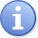Actually, there are a couple of more sophisticated techniques for implementing the Priority Queue ADT. The Fibonacci heap supports `add` in O(1) time and `remove` in O(log n) time. And there is the pair heap, which experiments indicate is faster than all other techniques, although researchers are still looking for a proof that its `add` method takes O(1) time. Both techniques are more complicated than we can investigate here, though.

## 8.3. Application: Sorting

One of the many applications of priority queues turns up in sorting. Here, we add all the elements of an array into a priority queue via the `add` method, and then we remove all elements via the `remove` method to copy them back into the array.

```public static void pQueueSort(Comparable[] data) {     PriorityQueue elts = new PriorityQueue(data.length);     for(int i = 0; i < data.length; i++) elts.add(data[i]);     for(int i = 0; i < data.length; i++) data[i] = elts.removeMin(); } ```

Both the selection sort and the insertion sort algorithms that we have already seen can be understood as implementations of this technique: For selection sort, our priority queue uses the unordered list representation. Initially, the queue holds all elements, but with each selection of the segment's minimum, the array segment representing the priority queue becomes one element shorter. For insertion sort, our priority queue uses the sorted list representation. The array segment holding the priority queue is initially just the first element alone, but then we add successive elements into the priority queue. Once we've completed removing the elements, the insertion sort algorithm returns them in the order they exist within the priority queue.

We can similarly use a heap to sort numbers, where we designate a portion of the array as the heap, from which we successively remove elements into the undesignated portion, to arrive at a completely sorted array. To do this, we will invert the traditional heap so that each node has a value that is larger than the values of its children, and each removal extracts the heap's maximum value. Our heap will be the first portion of the array; the first phase will be to add all the elements to the heap.Heap segment starts with first element only.Then we grow it to the first two elements.Then the first three elements.Then the first four elements.… until the segment covers the entire array.In the second phase, we successively remove the maximum value and place it into the appropriate place of the array.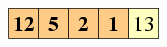Remove the heap's maximum into the final slot.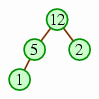The next removal goes into the slot before that.And the next goes into the slot before that.… until the heap has only one element left.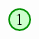This is the idea underlying the Heapsort algorithm, although Heapsort is slightly different from the above algorithm in that it uses a different algorithm for the first phase of building the heap: Rather than taking the approach of successively inserting each element into a single heap, it builds a heap from the bottom up, starting with n / 2 one-node heaps and then merging them.We start with the last n / 2 elements being one-node heaps.Then we take the next position (where there is a 5), let the two heaps below it be subtrees, and trickle the value downward until we have a heap in that position.We repeat the process until there is one heap left.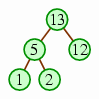We call this process heapifying, and it is used in the `heapSort` implementation of Figure 8.4.

Figure 8.4: The `heapSort` method.

```public static void heapSort(int[] data) {     // heapify     int size = data.length; // length of heap segment of array     for(int i = (size - 2) / 2; i >= 0; i--) {         trickleDown(data, size, i, data[i]);     }     // remove successive items out of heap     while(size > 1) {         size--;         int toTrickle = data[size];         data[size] = data;         trickleDown(data, size, 0, toTrickle);     } } private static void trickleDown(int[] data, int size, int pos, int toTrickle) {     while(true) {         int less = 2 * pos + 1;         if(less >= size) break;         if(less + 1 < size && data[less + 1] > data[less]) less++;         if(toTrickle > data[less]) break;         data[pos] = data[less];         pos = less;     }     data[pos] = toTrickle; } ```

The advantage of the heapify process is that it is somewhat faster. Creating a heap by adding n elements successively into a single heap takes O(n log n) time, since adding an element into a heap of k elements takes O(log k) time.

log 1 + log 2 + log 3 + … + log (n − 1) + log n ≤ n log n

However, we can get a better bound with heapifying, because the first n / 2 elements take zero time, the next n / 4 elements can go down only one level, the next n / 8 elements can go down at most two levels, and so on.

0 ⋅ (n/2) + 1 ⋅ (n/4) + 2 ⋅ (n/8) + 3 ⋅ (n/16) + … + (log2 n) ⋅ 1

It turns out that this sequence can be bounded by n, and so the heapify process takes O(n) time. Using this process, the first phase of Heapsort takes O(n) time. The second phase still takes O(n log n) time, so it does not change the overall speed bound, but the algorithm is marginally faster.The fact that the sequence can be bounded by n is illustrated by the following table.

 (n/4) + (n/8) + (n/16) + … + 1 ≤ n/2 (n/8) + (n/16) + … + 1 ≤ n/4 (n/16) + … + 1 ≤ n/8 : : : 1 ≤ 1 1 ⋅ (n/4) + 2 ⋅ (n/8) + 3 ⋅ (n/16) + … + (log2 n) ⋅ 1 ≤ n

For each row of the table but the final row, the left side of the inequality contains a geometric sequence, which is approximated on the right. The final row represents the sum of all the rows above: Each column of the final inequality's left side represents the sum of the column entries above. Note that this final inequality's left side matches the expression for the speed of the heapify process. Because it matches the sum of the inequalities above the bottom row, it can be approximated by the sum of the approximations for each of these inequalities, and the sum of these approximations (the table's rightmost column) is itself a geometric sequence that can be approximated by n.

In practice, though they both take O(n log n) time, Heapsort is not quite as fast as Quicksort, because it moves elements around fairly often. Nonetheless, it is an interesting alternative that is quite efficient.

## 8.4. Application: Shortest path

For another interesting application of priority queues, we consider the problem of finding the shortest distance between two cities on a network of roads. Suppose, for example, that we have the map in Figure 8.5 of roads in the Arkansas Ozarks, and we want to determine the length of the best route from Conway to Mountain Home.

Figure 8.5: An example map of roads in the Arkansas Ozarks.(Mathematicians and computer scientists call such a network of points and links a graph. Problems involving graphs, including the shortest-path problem, inspire a large number of interesting algorithms.)

### 8.4.1. Dijkstra's algorithm

One of the best possible techniques for computing this distance is known as Dijkstra's algorithm. For this algorithm, we maintain a collection called known of towns whose distances from the beginning are known. Initially, this collection is empty. We gradually grow this collection, starting from Conway, until it includes our destination, Mountain Home. To grow the collection, we'll maintain another collection fringe, which contains cities where we know a route to each from Conway, paired with the length of that route; they aren't in known yet, though, because we may later discover a shorter route to them. With each step, we'll remove the candidate with the shortest distance in fringe, add it to known, and add any neighbors not already in known into fringe. You can think of this as working like a bubble (represented by known) that starts at the starting location and grows to includes cities progressively farther away until it includes the destination city.

The following pseudocode illustrates this algorithm.

Let known be empty.
Let fringe contain the element (Conway, 0) only.
while Mountain Home ∉ known, do:
Remove city c with smallest distance d from fringe.
if c ∉ known, then:
for each neighbor b of c, do:
if b ∉ known, then:
Let x be distance from c to b.
Add (bd + x) to fringe.

The table of Figure 8.6 illustrates how this would work with the map of Figure 8.5. In it, you can see why our algorithm does not always immediately place cities into known, instead saving them in fringe: In processing Heber Springs, we place Mountain View into fringe. But we discover a shorter route to Mountain View in the next step, when we visit Clinton.

Figure 8.6: A trace of Dijkstra's algorithm on the map of Figure 8.5.

removed (cd) known fringe
initial (Conway, 0)
(Conway, 0) (Conway, 0) (Clinton, 43), (Heber Springs, 42), (Russellville, 49)
(Heber Springs, 42) (Conway, 0), (Heber Springs, 42) (Clinton, 43), (Mountain View, 82), (Russellville, 49)
(Clinton, 43) (Clinton, 43), (Conway, 0), (Heber Springs, 42) (Mountain View, 81), (Mountain View, 82), (Pindall, 91), (Russellville, 49)
(Russellville, 49) (Clinton, 43), (Conway, 0), (Heber Springs, 42), (Russellville, 49) (Mountain View, 81), (Mountain View, 82), (Pindall, 91), (Pindall, 140)
(Mountain View, 81) (Clinton, 43), (Conway, 0), (Heber Springs, 42), (Mountain View, 81), (Russellville, 49) (Mountain Home, 134), (Mountain View, 82), (Pindall, 91), (Pindall, 140)
(Mountain View, 82) no change (Mountain Home, 134), (Pindall, 91), (Pindall, 140)
(Pindall, 91) (Clinton, 43), (Conway, 0), (Heber Springs, 42), (Mountain View, 81), (Pindall, 91), (Russellville, 49) (Mountain Home, 134), (Mountain Home, 138), (Pindall, 140)
(Mountain Home, 134) (Clinton, 43), (Conway, 0), (Heber Springs, 42), (Mountain Home, 134), (Mountain View, 81), (Pindall, 91), (Russellville, 49) (Mountain Home, 138), (Pindall, 140)

To implement Dijkstra's algorithm, we would need to choose the appropriate data structure for both known and fringe. The only operations on known are `add` and `contains`, so the appropriate ADT for it would be the Set ADT; and the HashSet would be suitable data structure. For fringe, the operations include `add` and `remove`, and so it represents an instance of the Priority Queue ADT; and a heap would be a suitable structure for it.

Using a hash table for known and a heap for fringe, we can ask: How much time will Dijkstra's algorithm take to find the best distance? We can try to measure this in terms of n, the number of cities. First, we need to determine the maximum size of fringe. An element b is added into fringe only when b is not in known and we are looking at a c that is in known — so we add an element into fringe only once per road. The number of roads cannot be larger than n², since there are at the most n roads coming from each of the n towns; thus fringe can never have more than n² elements in it.

The inner loop, then, which steps through all the neighbors of c, involves a test for containment within the hash table known — taking O(1) time — and an addition into the heap fringe — taking O(log (n²)) = O(2 log n) = O(log n) time. Thus, each iteration of the inner loop takes a total of O(log n) time. Each city c may have as many as n neighbors, so the inner loop may have as many as n iterations per c, for a total of O(n log n) time.

We will perform this inner loop at most once per city, since we do it only when c is not in known, and then we would also add c into known to ensure that it will not occur again. Thus, the total amount of time spent on the inner loop over the course of the algorithm is O(n² log n). Aside from our time for the inner loop, each iteration of the outer loop takes O(log n) time, since it involves a O(log n) removal from the fringe heap, a O(1) containment test, and a possible O(1) addition to the known hash table. The outer loop may have as many as n² iterations, once for each element added into fringe, for a total of O(n² log n) time spent on the outer loop, neglecting the time spent on the inner loop. The time spent in inner loop overall is also O(n² log n), so the total time is O(n² log n). In terms of big-O notation, Dijkstra's algorithm is essentially the fastest known algorithm for finding the shortest distance between two points on a map.

(Actually, the method presented here is somewhat worse than the best implementation. Using a Fibonacci heap and a slightly modified version of what was presented here, Dijkstra's algorithm provides a bound of O(m + n log n), where m is the number of roads.)

### 8.4.2. A* search algorithm

Though Dijkstra's algorithm provides the best theoretic bound known, we can still hope for some practical improvement. In particular, Dijkstra's algorithm will search in a circle inflating outward from the starting point; in maps of the real world, it makes little sense to waste much time with the side of the circle that is farther away from the destination. It would be better if the bubble would inflate more quickly toward the destination. This is the idea underlying the A* search algorithm.

With A* search, we require some heuristic function that maps cities to distances — in particular, each city is associated with some underestimate of the true distance from that city to the destination that we can compute quickly. (The requirement … that we can compute quickly prevents us from using the true distance. If we could already determine that quickly, there would be no point in trying to solve the problem.) For a map, the natural heuristic function would be the crow's distance — that is, the length of a straight-line flight from the city in question to the destination. Figure 8.7 enhances our earlier map with some heuristic values.

Figure 8.7: A map with heuristic values.cityh(city)
Clinton 52
Conway 86
Heber Springs 63
Mountain Home 0
Mountain View 36
Pindall 34
Russellville 88

Once we have a heuristic function, then we'll associate with each city c in fringe an estimate of the distance from the start location to the destination via c — we'll add the known distance from the start location to c and the heuristic distance from c to the destination. Below is the modified algorithm; the notation h(x) represents the heuristic value for the city x.

Let known be empty.
Let fringe contain the element (Conway, 0, h(Conway)) only.
while Mountain Home ∉ known, do:
Remove element (cde) from fringe where e is smallest.
if c ∉ known, then:
for each neighbor b of c, do:
if b ∉ known, then:
Let x be distance from c to b.
Add (bd + xd + x + h(b)) to fringe.

Figure 8.8 illustrates how the A* search algorithm would perform on our earlier problem, using the heuristic function listed in Figure 8.7.

Figure 8.8: A trace of the A* search algorithm on the map of Figure 8.7.

removed (cde) known fringe
initial (Conway, 0, 52)
(Conway, 0, 52) (Conway, 0) (Clinton, 43, 95), (Heber Springs, 42, 105), (Russellville, 49, 137)
(Clinton, 43, 95) (Conway, 0), (Clinton, 43) (Heber Springs, 42, 105), (Russellville, 49, 137), (Mountain View, 81, 117), (Pindall, 91, 125)
(Heber Springs, 42, 105)  (Conway, 0), (Clinton, 43), (Heber Springs, 42) (Russellville, 49, 137), (Mountain View, 81, 117), (Pindall, 91, 125), (Mountain View, 82, 118)
(Mountain View, 81, 117) (Clinton, 43), (Conway, 0), (Heber Springs, 42), (Mountain View, 81) (Russellville, 49, 137), (Pindall, 91, 125), (Mountain View, 82, 118), (Mountain Home, 135, 135)
(Mountain View, 82, 118) no change (Russellville, 49, 137), (Pindall, 91, 125), (Mountain Home, 135, 135)
(Pindall, 91, 125) (Clinton, 43), (Conway, 0), (Heber Springs, 42), (Mountain View, 81), (Pindall, 125) (Russellville, 49, 137), (Mountain Home, 135, 135), (Mountain Home, 138, 138)
(Mountain Home, 135, 135) (Clinton, 43), (Conway, 0), (Heber Springs, 42), (Mountain View, 81), (Pindall, 125), (Mountain Home, 135) (Russellville, 49, 137), (Mountain Home, 138, 138)

You can see that it takes slightly fewer iterations that before; in particular, it concluded early on that there was little point in examining Russellville, even if it was close to Conway, because the heuristic function indicated that it was so far from Mountain Home. If we were doing A* on a more complete map, including cities south of Conway, the difference between the real performance of Dijkstra's algorithm and the A* algorithm would be even more noticable.

Using the same analysis as for Dijkstra's algorithm, the big-O bound on the A* search algorithm's performance is the same O(n² log n). Without allowing room for some quantification of the heuristic function's accuracy (which would be quite complicated), we cannot improve on this bound.

Dijkstra's algorithm and A* search algorithm demonstrate a sophisticated usage of the analysis we have seen in this book, applied to a useful problem. Our analysis involved two data structures — hash tables and heaps — of the several important data structures we have seen. This is just one of the many important, practical, and interesting problems where the analytic tools and the knowledge of data structures that we have investigated have proven useful.